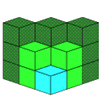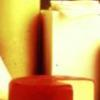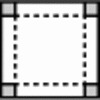#### You may also likeIf you had 36 cubes, what different cuboids could you make?### The Big Cheese

Investigate the area of 'slices' cut off this cube of cheese. What would happen if you had different-sized block of cheese to start with?### Making Boxes

Cut differently-sized square corners from a square piece of paper to make boxes without lids. Do they all have the same volume?

# All Wrapped Up

##### Age 7 to 11Challenge Level

This was a tricky problem, but many of you tackled it successfully. Your solutions depended on whether you assumed that you could cut the paper or not. Rachel from Charter Primary said:

I solved this problem by drawing a net.

The length of my cuboid was $38 \; \text{cm}$. The width of my cuboid was 14cm. The depth of my cuboid was $1\; \text{cm}$.

I tried to make the unused space as small as possible.

So, Rachel's solution kept the paper in one piece (as you would usually do when you wrap a present, for example).

This gives a cuboid with the maximum possible surface area ($1168 \;\text{cm}^2$).

I wonder how you arrived at this solution, Rachel? How many differently-sized cuboids did you try before you discovered this was the largest? Rohaan from Longbay Primary looked at it in a different way, assuming that you could cut the paper:

We decided to find a cuboid that that had a surface area that matched with the wrapping paper ($1200 \; \text{cm}^2$).

We made a starting estimate of a cuboid that was $1\times20\times20$. It had a surface area of $880 \; \text{cm}^2$.

Then we thought there could be a bigger cuboid that would fit. We wondered by how much the cuboid's surface area would go up if we changed its measurements from $1\times20\times20$ to $2\times20\times20$. It went up $80 \; \text{cm}^2$.

We thought if we changed it to $3\times20\times20$ it would go up by $80 \; \text{cm}^2$ again. It did. We went up until we reached $5\times20\times20$ which had a surface area of exactly $1200 \; \text{cm}^2$. It matched the surface area of the wrapping paper.

Just to make sure it fitted, we drew up the surface area ($1200 \; \text{cm}^2$) on a piece of A3 paper. It fitted!

Did anyone try to find the cuboid with the largest volume that could be wrapped up in this paper? That's another challenge for you!